# Surveying Mod3_Contouring.pptx

23. Jan 2023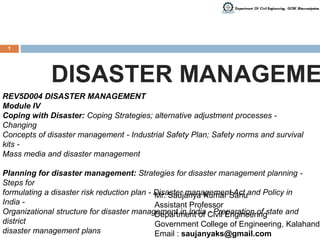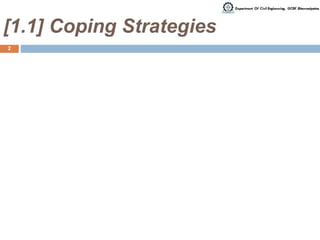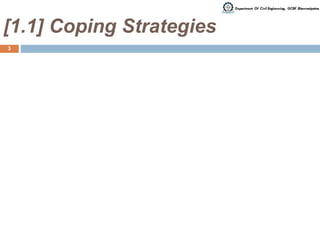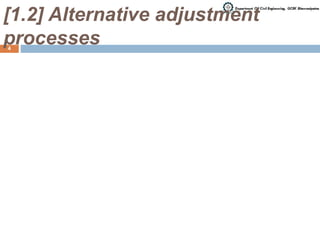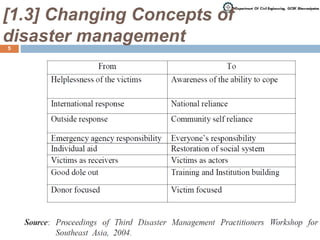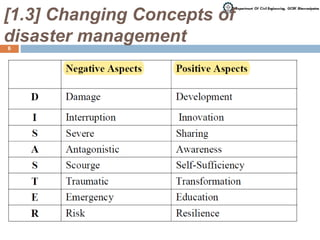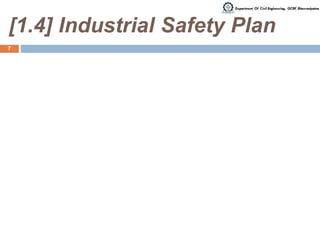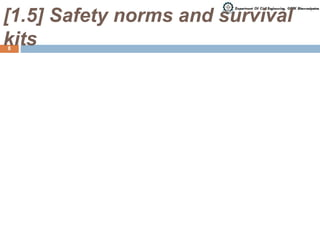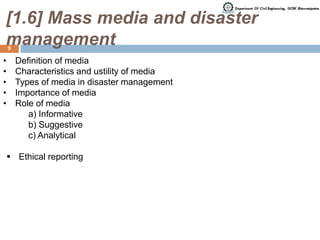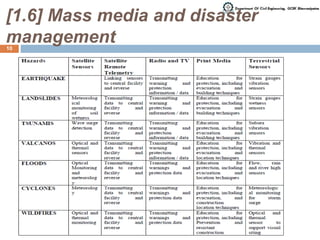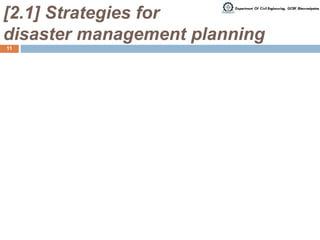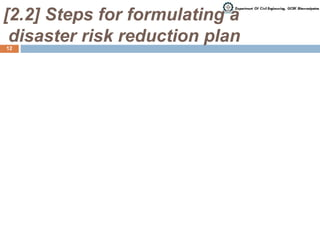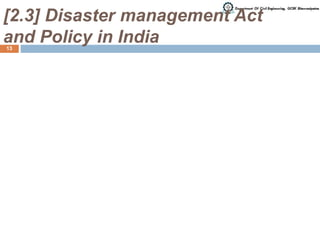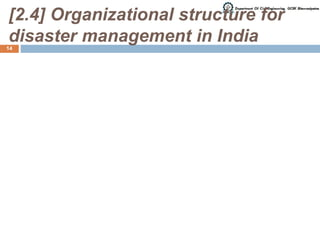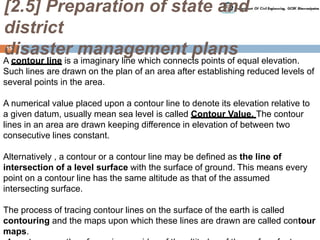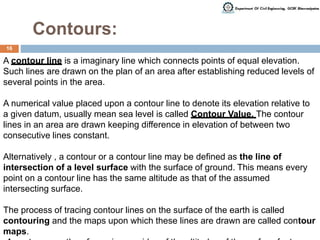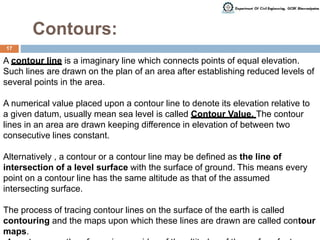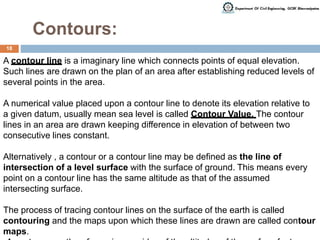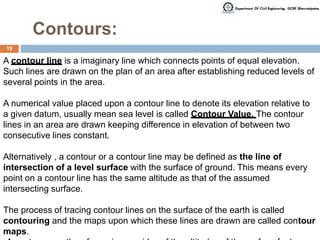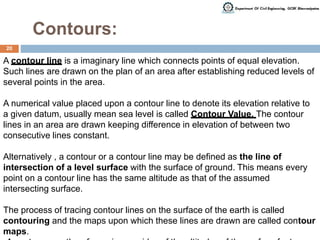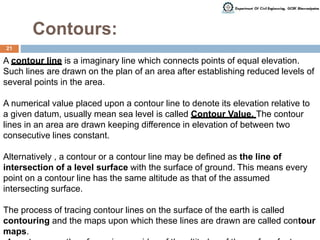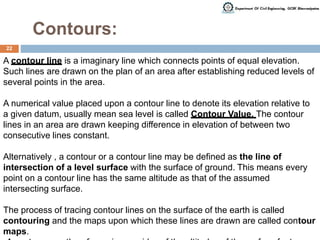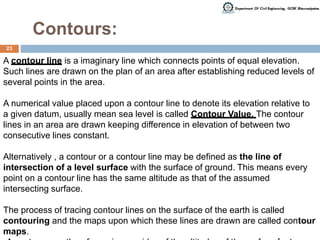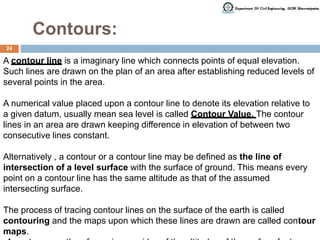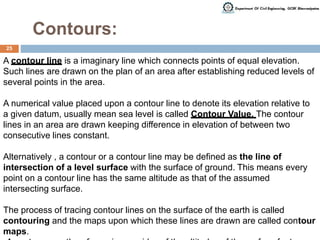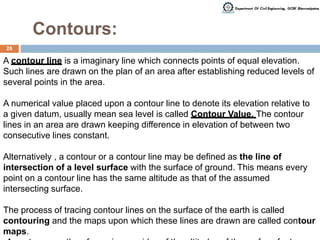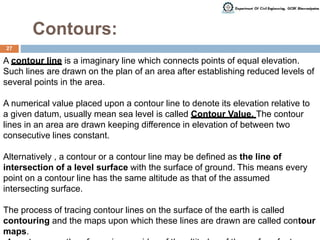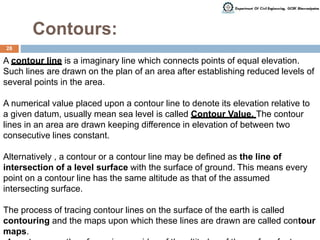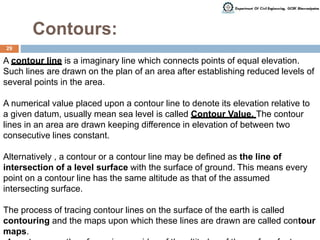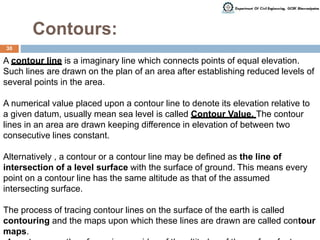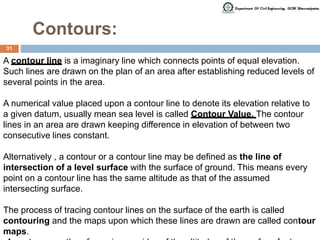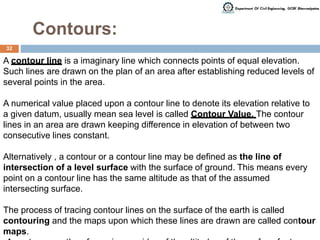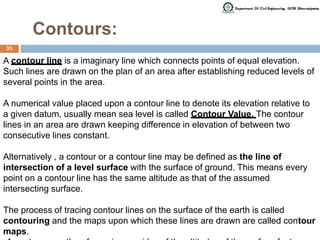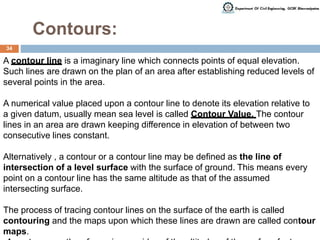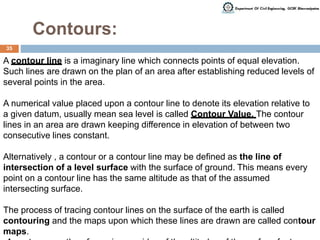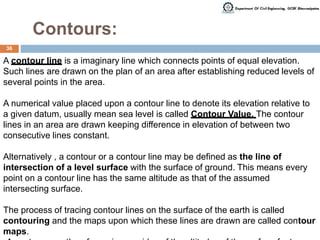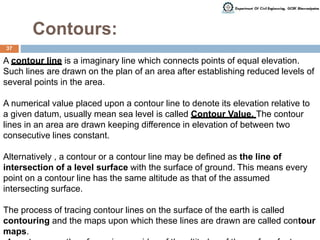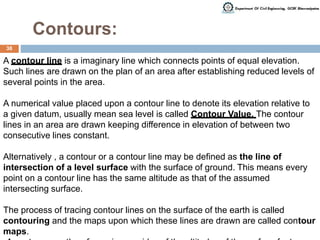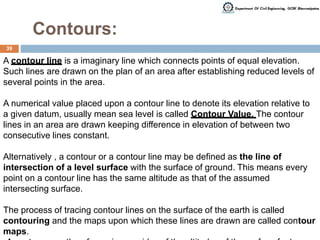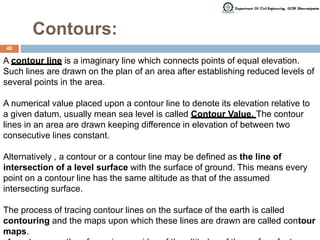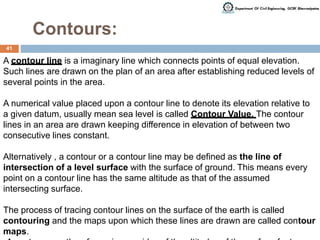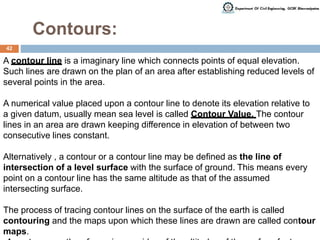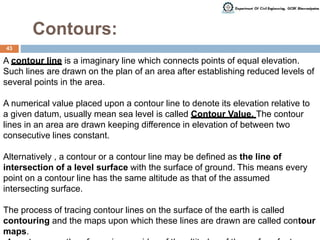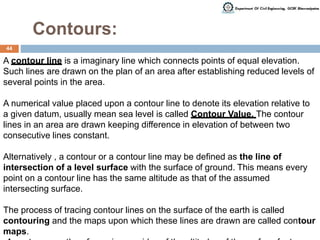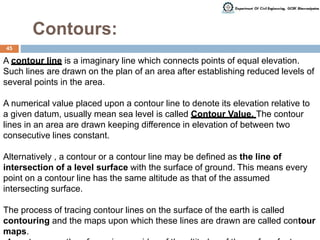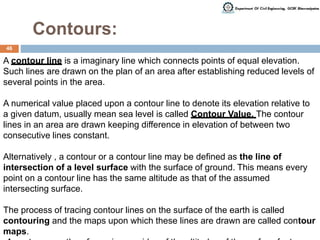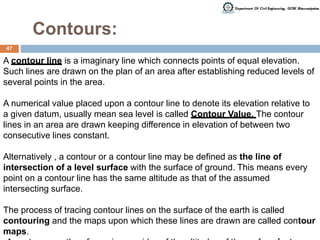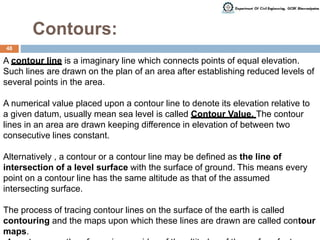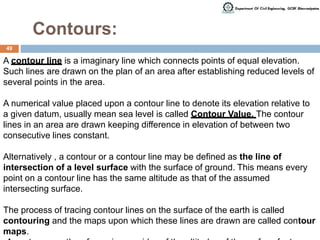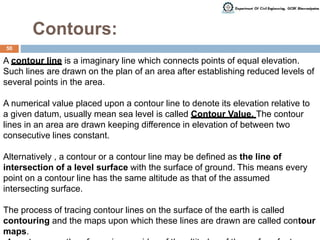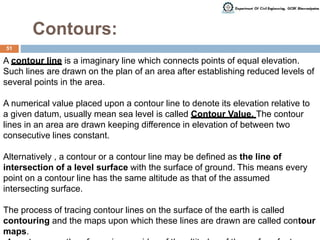1 von 51

### Surveying Mod3_Contouring.pptx

• 1. 1 REV5D004 DISASTER MANAGEMENT Module IV Coping with Disaster: Coping Strategies; alternative adjustment processes - Changing Concepts of disaster management - Industrial Safety Plan; Safety norms and survival kits - Mass media and disaster management Planning for disaster management: Strategies for disaster management planning - Steps for formulating a disaster risk reduction plan - Disaster management Act and Policy in India - Organizational structure for disaster management in India - Preparation of state and district disaster management plans DISASTER MANAGEME Mr. Saujanya Kumar Sahu Assistant Professor Department of Civil Engineering Government College of Engineering, Kalahandi Email : saujanyaks@gmail.com
• 2. [1.1] Coping Strategies 2
• 3. [1.1] Coping Strategies 3
• 4. [1.2] Alternative adjustment processes 4
• 5. [1.3] Changing Concepts of disaster management 5
• 6. [1.3] Changing Concepts of disaster management 6
• 7. [1.4] Industrial Safety Plan 7
• 8. [1.5] Safety norms and survival kits 8
• 9. [1.6] Mass media and disaster management • Definition of media • Characteristics and ustility of media • Types of media in disaster management • Importance of media • Role of media a) Informative b) Suggestive c) Analytical  Ethical reporting 9
• 10. [1.6] Mass media and disaster management 10
• 11. [2.1] Strategies for disaster management planning 11
• 12. [2.2] Steps for formulating a disaster risk reduction plan 12
• 13. [2.3] Disaster management Act and Policy in India 13
• 14. [2.4] Organizational structure for disaster management in India 14
• 15. [2.5] Preparation of state and district disaster management plans A contour line is a imaginary line which connects points of equal elevation. Such lines are drawn on the plan of an area after establishing reduced levels of several points in the area. A numerical value placed upon a contour line to denote its elevation relative to a given datum, usually mean sea level is called Contour Value. The contour lines in an area are drawn keeping difference in elevation of between two consecutive lines constant. Alternatively , a contour or a contour line may be defined as the line of intersection of a level surface with the surface of ground. This means every point on a contour line has the same altitude as that of the assumed intersecting surface. The process of tracing contour lines on the surface of the earth is called contouring and the maps upon which these lines are drawn are called contour maps. 15
• 16. Contours: A contour line is a imaginary line which connects points of equal elevation. Such lines are drawn on the plan of an area after establishing reduced levels of several points in the area. A numerical value placed upon a contour line to denote its elevation relative to a given datum, usually mean sea level is called Contour Value. The contour lines in an area are drawn keeping difference in elevation of between two consecutive lines constant. Alternatively , a contour or a contour line may be defined as the line of intersection of a level surface with the surface of ground. This means every point on a contour line has the same altitude as that of the assumed intersecting surface. The process of tracing contour lines on the surface of the earth is called contouring and the maps upon which these lines are drawn are called contour maps. 16
• 17. Contours: A contour line is a imaginary line which connects points of equal elevation. Such lines are drawn on the plan of an area after establishing reduced levels of several points in the area. A numerical value placed upon a contour line to denote its elevation relative to a given datum, usually mean sea level is called Contour Value. The contour lines in an area are drawn keeping difference in elevation of between two consecutive lines constant. Alternatively , a contour or a contour line may be defined as the line of intersection of a level surface with the surface of ground. This means every point on a contour line has the same altitude as that of the assumed intersecting surface. The process of tracing contour lines on the surface of the earth is called contouring and the maps upon which these lines are drawn are called contour maps. 17
• 18. Contours: A contour line is a imaginary line which connects points of equal elevation. Such lines are drawn on the plan of an area after establishing reduced levels of several points in the area. A numerical value placed upon a contour line to denote its elevation relative to a given datum, usually mean sea level is called Contour Value. The contour lines in an area are drawn keeping difference in elevation of between two consecutive lines constant. Alternatively , a contour or a contour line may be defined as the line of intersection of a level surface with the surface of ground. This means every point on a contour line has the same altitude as that of the assumed intersecting surface. The process of tracing contour lines on the surface of the earth is called contouring and the maps upon which these lines are drawn are called contour maps. 18
• 19. Contours: A contour line is a imaginary line which connects points of equal elevation. Such lines are drawn on the plan of an area after establishing reduced levels of several points in the area. A numerical value placed upon a contour line to denote its elevation relative to a given datum, usually mean sea level is called Contour Value. The contour lines in an area are drawn keeping difference in elevation of between two consecutive lines constant. Alternatively , a contour or a contour line may be defined as the line of intersection of a level surface with the surface of ground. This means every point on a contour line has the same altitude as that of the assumed intersecting surface. The process of tracing contour lines on the surface of the earth is called contouring and the maps upon which these lines are drawn are called contour maps. 19
• 20. Contours: A contour line is a imaginary line which connects points of equal elevation. Such lines are drawn on the plan of an area after establishing reduced levels of several points in the area. A numerical value placed upon a contour line to denote its elevation relative to a given datum, usually mean sea level is called Contour Value. The contour lines in an area are drawn keeping difference in elevation of between two consecutive lines constant. Alternatively , a contour or a contour line may be defined as the line of intersection of a level surface with the surface of ground. This means every point on a contour line has the same altitude as that of the assumed intersecting surface. The process of tracing contour lines on the surface of the earth is called contouring and the maps upon which these lines are drawn are called contour maps. 20
• 21. Contours: A contour line is a imaginary line which connects points of equal elevation. Such lines are drawn on the plan of an area after establishing reduced levels of several points in the area. A numerical value placed upon a contour line to denote its elevation relative to a given datum, usually mean sea level is called Contour Value. The contour lines in an area are drawn keeping difference in elevation of between two consecutive lines constant. Alternatively , a contour or a contour line may be defined as the line of intersection of a level surface with the surface of ground. This means every point on a contour line has the same altitude as that of the assumed intersecting surface. The process of tracing contour lines on the surface of the earth is called contouring and the maps upon which these lines are drawn are called contour maps. 21
• 22. Contours: A contour line is a imaginary line which connects points of equal elevation. Such lines are drawn on the plan of an area after establishing reduced levels of several points in the area. A numerical value placed upon a contour line to denote its elevation relative to a given datum, usually mean sea level is called Contour Value. The contour lines in an area are drawn keeping difference in elevation of between two consecutive lines constant. Alternatively , a contour or a contour line may be defined as the line of intersection of a level surface with the surface of ground. This means every point on a contour line has the same altitude as that of the assumed intersecting surface. The process of tracing contour lines on the surface of the earth is called contouring and the maps upon which these lines are drawn are called contour maps. 22
• 23. Contours: A contour line is a imaginary line which connects points of equal elevation. Such lines are drawn on the plan of an area after establishing reduced levels of several points in the area. A numerical value placed upon a contour line to denote its elevation relative to a given datum, usually mean sea level is called Contour Value. The contour lines in an area are drawn keeping difference in elevation of between two consecutive lines constant. Alternatively , a contour or a contour line may be defined as the line of intersection of a level surface with the surface of ground. This means every point on a contour line has the same altitude as that of the assumed intersecting surface. The process of tracing contour lines on the surface of the earth is called contouring and the maps upon which these lines are drawn are called contour maps. 23
• 24. Contours: A contour line is a imaginary line which connects points of equal elevation. Such lines are drawn on the plan of an area after establishing reduced levels of several points in the area. A numerical value placed upon a contour line to denote its elevation relative to a given datum, usually mean sea level is called Contour Value. The contour lines in an area are drawn keeping difference in elevation of between two consecutive lines constant. Alternatively , a contour or a contour line may be defined as the line of intersection of a level surface with the surface of ground. This means every point on a contour line has the same altitude as that of the assumed intersecting surface. The process of tracing contour lines on the surface of the earth is called contouring and the maps upon which these lines are drawn are called contour maps. 24
• 25. Contours: A contour line is a imaginary line which connects points of equal elevation. Such lines are drawn on the plan of an area after establishing reduced levels of several points in the area. A numerical value placed upon a contour line to denote its elevation relative to a given datum, usually mean sea level is called Contour Value. The contour lines in an area are drawn keeping difference in elevation of between two consecutive lines constant. Alternatively , a contour or a contour line may be defined as the line of intersection of a level surface with the surface of ground. This means every point on a contour line has the same altitude as that of the assumed intersecting surface. The process of tracing contour lines on the surface of the earth is called contouring and the maps upon which these lines are drawn are called contour maps. 25
• 26. Contours: A contour line is a imaginary line which connects points of equal elevation. Such lines are drawn on the plan of an area after establishing reduced levels of several points in the area. A numerical value placed upon a contour line to denote its elevation relative to a given datum, usually mean sea level is called Contour Value. The contour lines in an area are drawn keeping difference in elevation of between two consecutive lines constant. Alternatively , a contour or a contour line may be defined as the line of intersection of a level surface with the surface of ground. This means every point on a contour line has the same altitude as that of the assumed intersecting surface. The process of tracing contour lines on the surface of the earth is called contouring and the maps upon which these lines are drawn are called contour maps. 26
• 27. Contours: A contour line is a imaginary line which connects points of equal elevation. Such lines are drawn on the plan of an area after establishing reduced levels of several points in the area. A numerical value placed upon a contour line to denote its elevation relative to a given datum, usually mean sea level is called Contour Value. The contour lines in an area are drawn keeping difference in elevation of between two consecutive lines constant. Alternatively , a contour or a contour line may be defined as the line of intersection of a level surface with the surface of ground. This means every point on a contour line has the same altitude as that of the assumed intersecting surface. The process of tracing contour lines on the surface of the earth is called contouring and the maps upon which these lines are drawn are called contour maps. 27
• 28. Contours: A contour line is a imaginary line which connects points of equal elevation. Such lines are drawn on the plan of an area after establishing reduced levels of several points in the area. A numerical value placed upon a contour line to denote its elevation relative to a given datum, usually mean sea level is called Contour Value. The contour lines in an area are drawn keeping difference in elevation of between two consecutive lines constant. Alternatively , a contour or a contour line may be defined as the line of intersection of a level surface with the surface of ground. This means every point on a contour line has the same altitude as that of the assumed intersecting surface. The process of tracing contour lines on the surface of the earth is called contouring and the maps upon which these lines are drawn are called contour maps. 28
• 29. Contours: A contour line is a imaginary line which connects points of equal elevation. Such lines are drawn on the plan of an area after establishing reduced levels of several points in the area. A numerical value placed upon a contour line to denote its elevation relative to a given datum, usually mean sea level is called Contour Value. The contour lines in an area are drawn keeping difference in elevation of between two consecutive lines constant. Alternatively , a contour or a contour line may be defined as the line of intersection of a level surface with the surface of ground. This means every point on a contour line has the same altitude as that of the assumed intersecting surface. The process of tracing contour lines on the surface of the earth is called contouring and the maps upon which these lines are drawn are called contour maps. 29
• 30. Contours: A contour line is a imaginary line which connects points of equal elevation. Such lines are drawn on the plan of an area after establishing reduced levels of several points in the area. A numerical value placed upon a contour line to denote its elevation relative to a given datum, usually mean sea level is called Contour Value. The contour lines in an area are drawn keeping difference in elevation of between two consecutive lines constant. Alternatively , a contour or a contour line may be defined as the line of intersection of a level surface with the surface of ground. This means every point on a contour line has the same altitude as that of the assumed intersecting surface. The process of tracing contour lines on the surface of the earth is called contouring and the maps upon which these lines are drawn are called contour maps. 30
• 31. Contours: A contour line is a imaginary line which connects points of equal elevation. Such lines are drawn on the plan of an area after establishing reduced levels of several points in the area. A numerical value placed upon a contour line to denote its elevation relative to a given datum, usually mean sea level is called Contour Value. The contour lines in an area are drawn keeping difference in elevation of between two consecutive lines constant. Alternatively , a contour or a contour line may be defined as the line of intersection of a level surface with the surface of ground. This means every point on a contour line has the same altitude as that of the assumed intersecting surface. The process of tracing contour lines on the surface of the earth is called contouring and the maps upon which these lines are drawn are called contour maps. 31
• 32. Contours: A contour line is a imaginary line which connects points of equal elevation. Such lines are drawn on the plan of an area after establishing reduced levels of several points in the area. A numerical value placed upon a contour line to denote its elevation relative to a given datum, usually mean sea level is called Contour Value. The contour lines in an area are drawn keeping difference in elevation of between two consecutive lines constant. Alternatively , a contour or a contour line may be defined as the line of intersection of a level surface with the surface of ground. This means every point on a contour line has the same altitude as that of the assumed intersecting surface. The process of tracing contour lines on the surface of the earth is called contouring and the maps upon which these lines are drawn are called contour maps. 32
• 33. Contours: A contour line is a imaginary line which connects points of equal elevation. Such lines are drawn on the plan of an area after establishing reduced levels of several points in the area. A numerical value placed upon a contour line to denote its elevation relative to a given datum, usually mean sea level is called Contour Value. The contour lines in an area are drawn keeping difference in elevation of between two consecutive lines constant. Alternatively , a contour or a contour line may be defined as the line of intersection of a level surface with the surface of ground. This means every point on a contour line has the same altitude as that of the assumed intersecting surface. The process of tracing contour lines on the surface of the earth is called contouring and the maps upon which these lines are drawn are called contour maps. 33
• 34. Contours: A contour line is a imaginary line which connects points of equal elevation. Such lines are drawn on the plan of an area after establishing reduced levels of several points in the area. A numerical value placed upon a contour line to denote its elevation relative to a given datum, usually mean sea level is called Contour Value. The contour lines in an area are drawn keeping difference in elevation of between two consecutive lines constant. Alternatively , a contour or a contour line may be defined as the line of intersection of a level surface with the surface of ground. This means every point on a contour line has the same altitude as that of the assumed intersecting surface. The process of tracing contour lines on the surface of the earth is called contouring and the maps upon which these lines are drawn are called contour maps. 34
• 35. Contours: A contour line is a imaginary line which connects points of equal elevation. Such lines are drawn on the plan of an area after establishing reduced levels of several points in the area. A numerical value placed upon a contour line to denote its elevation relative to a given datum, usually mean sea level is called Contour Value. The contour lines in an area are drawn keeping difference in elevation of between two consecutive lines constant. Alternatively , a contour or a contour line may be defined as the line of intersection of a level surface with the surface of ground. This means every point on a contour line has the same altitude as that of the assumed intersecting surface. The process of tracing contour lines on the surface of the earth is called contouring and the maps upon which these lines are drawn are called contour maps. 35
• 36. Contours: A contour line is a imaginary line which connects points of equal elevation. Such lines are drawn on the plan of an area after establishing reduced levels of several points in the area. A numerical value placed upon a contour line to denote its elevation relative to a given datum, usually mean sea level is called Contour Value. The contour lines in an area are drawn keeping difference in elevation of between two consecutive lines constant. Alternatively , a contour or a contour line may be defined as the line of intersection of a level surface with the surface of ground. This means every point on a contour line has the same altitude as that of the assumed intersecting surface. The process of tracing contour lines on the surface of the earth is called contouring and the maps upon which these lines are drawn are called contour maps. 36
• 37. Contours: A contour line is a imaginary line which connects points of equal elevation. Such lines are drawn on the plan of an area after establishing reduced levels of several points in the area. A numerical value placed upon a contour line to denote its elevation relative to a given datum, usually mean sea level is called Contour Value. The contour lines in an area are drawn keeping difference in elevation of between two consecutive lines constant. Alternatively , a contour or a contour line may be defined as the line of intersection of a level surface with the surface of ground. This means every point on a contour line has the same altitude as that of the assumed intersecting surface. The process of tracing contour lines on the surface of the earth is called contouring and the maps upon which these lines are drawn are called contour maps. 37
• 38. Contours: A contour line is a imaginary line which connects points of equal elevation. Such lines are drawn on the plan of an area after establishing reduced levels of several points in the area. A numerical value placed upon a contour line to denote its elevation relative to a given datum, usually mean sea level is called Contour Value. The contour lines in an area are drawn keeping difference in elevation of between two consecutive lines constant. Alternatively , a contour or a contour line may be defined as the line of intersection of a level surface with the surface of ground. This means every point on a contour line has the same altitude as that of the assumed intersecting surface. The process of tracing contour lines on the surface of the earth is called contouring and the maps upon which these lines are drawn are called contour maps. 38
• 39. Contours: A contour line is a imaginary line which connects points of equal elevation. Such lines are drawn on the plan of an area after establishing reduced levels of several points in the area. A numerical value placed upon a contour line to denote its elevation relative to a given datum, usually mean sea level is called Contour Value. The contour lines in an area are drawn keeping difference in elevation of between two consecutive lines constant. Alternatively , a contour or a contour line may be defined as the line of intersection of a level surface with the surface of ground. This means every point on a contour line has the same altitude as that of the assumed intersecting surface. The process of tracing contour lines on the surface of the earth is called contouring and the maps upon which these lines are drawn are called contour maps. 39
• 40. Contours: A contour line is a imaginary line which connects points of equal elevation. Such lines are drawn on the plan of an area after establishing reduced levels of several points in the area. A numerical value placed upon a contour line to denote its elevation relative to a given datum, usually mean sea level is called Contour Value. The contour lines in an area are drawn keeping difference in elevation of between two consecutive lines constant. Alternatively , a contour or a contour line may be defined as the line of intersection of a level surface with the surface of ground. This means every point on a contour line has the same altitude as that of the assumed intersecting surface. The process of tracing contour lines on the surface of the earth is called contouring and the maps upon which these lines are drawn are called contour maps. 40
• 41. Contours: A contour line is a imaginary line which connects points of equal elevation. Such lines are drawn on the plan of an area after establishing reduced levels of several points in the area. A numerical value placed upon a contour line to denote its elevation relative to a given datum, usually mean sea level is called Contour Value. The contour lines in an area are drawn keeping difference in elevation of between two consecutive lines constant. Alternatively , a contour or a contour line may be defined as the line of intersection of a level surface with the surface of ground. This means every point on a contour line has the same altitude as that of the assumed intersecting surface. The process of tracing contour lines on the surface of the earth is called contouring and the maps upon which these lines are drawn are called contour maps. 41
• 42. Contours: A contour line is a imaginary line which connects points of equal elevation. Such lines are drawn on the plan of an area after establishing reduced levels of several points in the area. A numerical value placed upon a contour line to denote its elevation relative to a given datum, usually mean sea level is called Contour Value. The contour lines in an area are drawn keeping difference in elevation of between two consecutive lines constant. Alternatively , a contour or a contour line may be defined as the line of intersection of a level surface with the surface of ground. This means every point on a contour line has the same altitude as that of the assumed intersecting surface. The process of tracing contour lines on the surface of the earth is called contouring and the maps upon which these lines are drawn are called contour maps. 42
• 43. Contours: A contour line is a imaginary line which connects points of equal elevation. Such lines are drawn on the plan of an area after establishing reduced levels of several points in the area. A numerical value placed upon a contour line to denote its elevation relative to a given datum, usually mean sea level is called Contour Value. The contour lines in an area are drawn keeping difference in elevation of between two consecutive lines constant. Alternatively , a contour or a contour line may be defined as the line of intersection of a level surface with the surface of ground. This means every point on a contour line has the same altitude as that of the assumed intersecting surface. The process of tracing contour lines on the surface of the earth is called contouring and the maps upon which these lines are drawn are called contour maps. 43
• 44. Contours: A contour line is a imaginary line which connects points of equal elevation. Such lines are drawn on the plan of an area after establishing reduced levels of several points in the area. A numerical value placed upon a contour line to denote its elevation relative to a given datum, usually mean sea level is called Contour Value. The contour lines in an area are drawn keeping difference in elevation of between two consecutive lines constant. Alternatively , a contour or a contour line may be defined as the line of intersection of a level surface with the surface of ground. This means every point on a contour line has the same altitude as that of the assumed intersecting surface. The process of tracing contour lines on the surface of the earth is called contouring and the maps upon which these lines are drawn are called contour maps. 44
• 45. Contours: A contour line is a imaginary line which connects points of equal elevation. Such lines are drawn on the plan of an area after establishing reduced levels of several points in the area. A numerical value placed upon a contour line to denote its elevation relative to a given datum, usually mean sea level is called Contour Value. The contour lines in an area are drawn keeping difference in elevation of between two consecutive lines constant. Alternatively , a contour or a contour line may be defined as the line of intersection of a level surface with the surface of ground. This means every point on a contour line has the same altitude as that of the assumed intersecting surface. The process of tracing contour lines on the surface of the earth is called contouring and the maps upon which these lines are drawn are called contour maps. 45
• 46. Contours: A contour line is a imaginary line which connects points of equal elevation. Such lines are drawn on the plan of an area after establishing reduced levels of several points in the area. A numerical value placed upon a contour line to denote its elevation relative to a given datum, usually mean sea level is called Contour Value. The contour lines in an area are drawn keeping difference in elevation of between two consecutive lines constant. Alternatively , a contour or a contour line may be defined as the line of intersection of a level surface with the surface of ground. This means every point on a contour line has the same altitude as that of the assumed intersecting surface. The process of tracing contour lines on the surface of the earth is called contouring and the maps upon which these lines are drawn are called contour maps. 46
• 47. Contours: A contour line is a imaginary line which connects points of equal elevation. Such lines are drawn on the plan of an area after establishing reduced levels of several points in the area. A numerical value placed upon a contour line to denote its elevation relative to a given datum, usually mean sea level is called Contour Value. The contour lines in an area are drawn keeping difference in elevation of between two consecutive lines constant. Alternatively , a contour or a contour line may be defined as the line of intersection of a level surface with the surface of ground. This means every point on a contour line has the same altitude as that of the assumed intersecting surface. The process of tracing contour lines on the surface of the earth is called contouring and the maps upon which these lines are drawn are called contour maps. 47
• 48. Contours: A contour line is a imaginary line which connects points of equal elevation. Such lines are drawn on the plan of an area after establishing reduced levels of several points in the area. A numerical value placed upon a contour line to denote its elevation relative to a given datum, usually mean sea level is called Contour Value. The contour lines in an area are drawn keeping difference in elevation of between two consecutive lines constant. Alternatively , a contour or a contour line may be defined as the line of intersection of a level surface with the surface of ground. This means every point on a contour line has the same altitude as that of the assumed intersecting surface. The process of tracing contour lines on the surface of the earth is called contouring and the maps upon which these lines are drawn are called contour maps. 48
• 49. Contours: A contour line is a imaginary line which connects points of equal elevation. Such lines are drawn on the plan of an area after establishing reduced levels of several points in the area. A numerical value placed upon a contour line to denote its elevation relative to a given datum, usually mean sea level is called Contour Value. The contour lines in an area are drawn keeping difference in elevation of between two consecutive lines constant. Alternatively , a contour or a contour line may be defined as the line of intersection of a level surface with the surface of ground. This means every point on a contour line has the same altitude as that of the assumed intersecting surface. The process of tracing contour lines on the surface of the earth is called contouring and the maps upon which these lines are drawn are called contour maps. 49
• 50. Contours: A contour line is a imaginary line which connects points of equal elevation. Such lines are drawn on the plan of an area after establishing reduced levels of several points in the area. A numerical value placed upon a contour line to denote its elevation relative to a given datum, usually mean sea level is called Contour Value. The contour lines in an area are drawn keeping difference in elevation of between two consecutive lines constant. Alternatively , a contour or a contour line may be defined as the line of intersection of a level surface with the surface of ground. This means every point on a contour line has the same altitude as that of the assumed intersecting surface. The process of tracing contour lines on the surface of the earth is called contouring and the maps upon which these lines are drawn are called contour maps. 50
• 51. Contours: A contour line is a imaginary line which connects points of equal elevation. Such lines are drawn on the plan of an area after establishing reduced levels of several points in the area. A numerical value placed upon a contour line to denote its elevation relative to a given datum, usually mean sea level is called Contour Value. The contour lines in an area are drawn keeping difference in elevation of between two consecutive lines constant. Alternatively , a contour or a contour line may be defined as the line of intersection of a level surface with the surface of ground. This means every point on a contour line has the same altitude as that of the assumed intersecting surface. The process of tracing contour lines on the surface of the earth is called contouring and the maps upon which these lines are drawn are called contour maps. 51